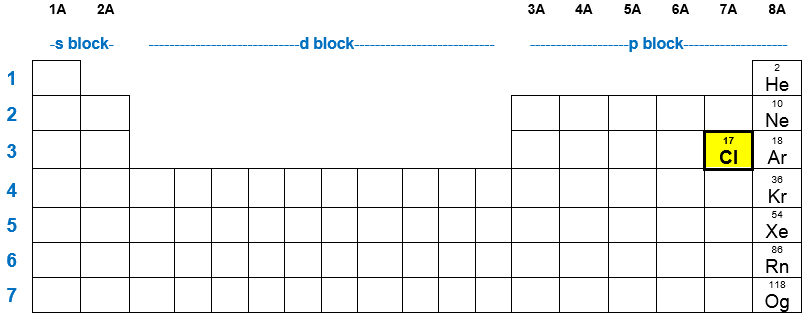# Problem: How many electrons in a Cl atom have an angular momentum quantum number of 0?a. 2b. 4c. 6d. 8e. 10

🤓 Based on our data, we think this question is relevant for Professor Shaka's class at UCI.

###### FREE Expert Solution

We are being asked to determine the number of electrons in a Cl atom that have an angular momentum quantum number ()  of 0. Let’s first write the electron configuration of a neutral Cl atom.

Neutral element:       Atomic number = # protons = # of electronsChlorine (Cl):

atomic number = 17 (refer to the periodic table)
# of electrons = 17
Distribute electrons in the orbitals:###### Problem Details

How many electrons in a Cl atom have an angular momentum quantum number of 0?

a. 2

b. 4

c. 6

d. 8

e. 10

What scientific concept do you need to know in order to solve this problem?

Our tutors have indicated that to solve this problem you will need to apply the Quantum Numbers concept. If you need more Quantum Numbers practice, you can also practice Quantum Numbers practice problems.

What is the difficulty of this problem?

Our tutors rated the difficulty ofHow many electrons in a Cl atom have an angular momentum qua...as medium difficulty.

How long does this problem take to solve?

Our expert Chemistry tutor, Jules took 1 minute and 36 seconds to solve this problem. You can follow their steps in the video explanation above.

What professor is this problem relevant for?

Based on our data, we think this problem is relevant for Professor Shaka's class at UCI.